09/07 15:18

# 一.Sobel算子

Sobel算子是一种用于边缘检测的离散微分算子，它结合了高斯平滑和微分求导。该算子用于计算图像明暗程度近似值，根据图像边缘旁边明暗程度把该区域内超过某个数的特定点记为边缘。Sobel算子在Prewitt算子的基础上增加了权重的概念，认为相邻点的距离远近对当前像素点的影响是不同的，距离越近的像素点对应当前像素的影响越大，从而实现图像锐化并突出边缘轮廓[1-4]。

Sobel算子的边缘定位更准确，常用于噪声较多、灰度渐变的图像。其算法模板如公式（1）所示，其中dx表示水平方向，dy表示垂直方向。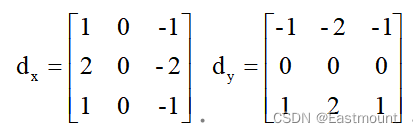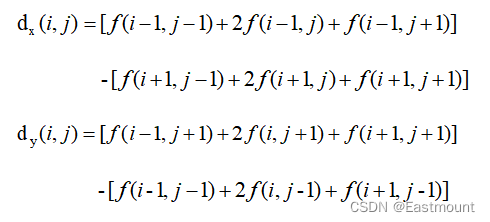Sobel算子像素的最终计算公式如下：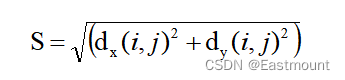Sobel算子根据像素点上下、左右邻点灰度加权差，在边缘处达到极值这一现象检测边缘。对噪声具有平滑作用，提供较为精确的边缘方向信息。因为Sobel算子结合了高斯平滑和微分求导（分化），因此结果会具有更多的抗噪性，当对精度要求不是很高时，Sobel算子是一种较为常用的边缘检测方法。

Python和OpenCV将Sobel算子封装在Sobel()函数中，其函数原型如下所示：

• dst = Sobel(src, ddepth, dx, dy[, dst[, ksize[, scale[, delta[, borderType]]]]])

– src表示输入图像

– dst表示输出的边缘图，其大小和通道数与输入图像相同

– ddepth表示目标图像所需的深度，针对不同的输入图像，输出目标图像有不同的深度

– dx表示x方向上的差分阶数，取值1或 0

– dy表示y方向上的差分阶数，取值1或0

– ksize表示Sobel算子的大小，其值必须是正数和奇数

– scale表示缩放导数的比例常数，默认情况下没有伸缩系数

– delta表示将结果存入目标图像之前，添加到结果中的可选增量值

– borderType表示边框模式，更多详细信息查阅BorderTypes

• dst = convertScaleAbs(src[, dst[, alpha[, beta]]])

– src表示原数组

– dst表示输出数组，深度为8位

– alpha表示比例因子

– beta表示原数组元素按比例缩放后添加的值

Sobel算子的实现代码如下所示。

# -*- coding: utf-8 -*-

# By:Eastmount

import cv2

import numpy as np

import matplotlib.pyplot as plt

#读取图像

lenna_img = cv2.cvtColor(img,cv2.COLOR_BGR2RGB)

#灰度化处理图像

grayImage = cv2.cvtColor(img, cv2.COLOR_BGR2GRAY)

#Sobel算子

x = cv2.Sobel(grayImage, cv2.CV_16S, 1, 0) #对x求一阶导

y = cv2.Sobel(grayImage, cv2.CV_16S, 0, 1) #对y求一阶导

absX = cv2.convertScaleAbs(x)

absY = cv2.convertScaleAbs(y)

Sobel = cv2.addWeighted(absX, 0.5, absY, 0.5, 0)

#用来正常显示中文标签

plt.rcParams['font.sans-serif']=['SimHei']

#显示图形

titles = ['原始图像', 'Sobel算子']

images = [lenna_img, Sobel]

for i in range(2):

plt.subplot(1,2,i+1), plt.imshow(images[i], 'gray')

plt.title(titles[i])

plt.xticks([]),plt.yticks([])

plt.show()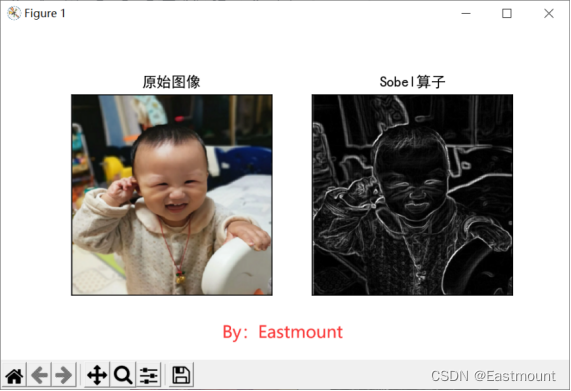# 二.Laplacian算子

• 判断图像中心像素灰度值与它周围其他像素的灰度值；
• 如果中心像素的灰度更高，则提升中心像素的灰度；
• 反之降低中心像素的灰度，从而实现图像锐化操作。Laplacian算子分为四邻域和八邻域，四邻域是对邻域中心像素的四方向求梯度，八邻域是对八方向求梯度。其中，四邻域模板如公式（5）所示：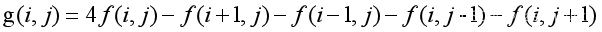Laplacian算子的八邻域模板如下：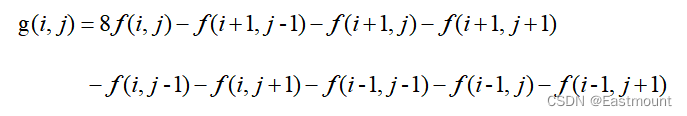Python和OpenCV将Laplacian算子封装在Laplacian()函数中，其函数原型如下所示：

• dst = Laplacian(src, ddepth[, dst[, ksize[, scale[, delta[, borderType]]]]])

– src表示输入图像

– dst表示输出的边缘图，其大小和通道数与输入图像相同

– ddepth表示目标图像所需的深度

– ksize表示用于计算二阶导数的滤波器的孔径大小，其值必须是正数和奇数，且默认值为1，更多详细信息查阅getDerivKernels

– scale表示计算拉普拉斯算子值的可选比例因子。默认值为1，更多详细信息查阅getDerivKernels

– delta表示将结果存入目标图像之前，添加到结果中的可选增量值，默认值为0

– borderType表示边框模式，更多详细信息查阅BorderTypes

• dst = convertScaleAbs(src[, dst[, alpha[, beta]]])

– src表示原数组

– dst表示输出数组，深度为8位

– alpha表示比例因子

– beta表示原数组元素按比例缩放后添加的值

# -*- coding: utf-8 -*-

# By:Eastmount

import cv2

import numpy as np

import matplotlib.pyplot as plt

#读取图像

lenna_img = cv2.cvtColor(img,cv2.COLOR_BGR2RGB)

#灰度化处理图像

grayImage = cv2.cvtColor(img, cv2.COLOR_BGR2GRAY)

#拉普拉斯算法

dst = cv2.Laplacian(grayImage, cv2.CV_16S, ksize = 3)

Laplacian = cv2.convertScaleAbs(dst)

#用来正常显示中文标签

plt.rcParams['font.sans-serif']=['SimHei']

#显示图形

titles = ['原始图像', 'Laplacian算子']

images = [lenna_img, Laplacian]

for i in range(2):

plt.subplot(1,2,i+1), plt.imshow(images[i], 'gray')

plt.title(titles[i])

plt.xticks([]),plt.yticks([])

plt.show()# -*- coding: utf-8 -*-

# By:Eastmount

import cv2

import numpy as np

import matplotlib.pyplot as plt

#读取图像

lenna_img = cv2.cvtColor(img, cv2.COLOR_BGR2RGB)

#灰度化处理图像

grayImage = cv2.cvtColor(img, cv2.COLOR_BGR2GRAY)

#高斯滤波

gaussianBlur = cv2.GaussianBlur(grayImage, (3,3), 0)

#阈值处理

ret, binary = cv2.threshold(gaussianBlur, 127, 255, cv2.THRESH_BINARY)

#Roberts算子

kernelx = np.array([[-1,0],[0,1]], dtype=int)

kernely = np.array([[0,-1],[1,0]], dtype=int)

x = cv2.filter2D(binary, cv2.CV_16S, kernelx)

y = cv2.filter2D(binary, cv2.CV_16S, kernely)

absX = cv2.convertScaleAbs(x)

absY = cv2.convertScaleAbs(y)

Roberts = cv2.addWeighted(absX, 0.5, absY, 0.5, 0)

#Prewitt算子

kernelx = np.array([[1,1,1],[0,0,0],[-1,-1,-1]], dtype=int)

kernely = np.array([[-1,0,1],[-1,0,1],[-1,0,1]], dtype=int)

x = cv2.filter2D(binary, cv2.CV_16S, kernelx)

y = cv2.filter2D(binary, cv2.CV_16S, kernely)

absX = cv2.convertScaleAbs(x)

absY = cv2.convertScaleAbs(y)

#Sobel算子

x = cv2.Sobel(binary, cv2.CV_16S, 1, 0)

y = cv2.Sobel(binary, cv2.CV_16S, 0, 1)

absX = cv2.convertScaleAbs(x)

absY = cv2.convertScaleAbs(y)

Sobel = cv2.addWeighted(absX, 0.5, absY, 0.5, 0)

#拉普拉斯算法

dst = cv2.Laplacian(binary, cv2.CV_16S, ksize = 3)

Laplacian = cv2.convertScaleAbs(dst)

#效果图

titles = ['Source Image', 'Binary Image', 'Roberts Image',

'Prewitt Image','Sobel Image', 'Laplacian Image']

images = [lenna_img, binary, Roberts, Prewitt, Sobel, Laplacian]

for i in np.arange(6):

plt.subplot(2,3,i+1),plt.imshow(images[i],'gray')

plt.title(titles[i])

plt.xticks([]),plt.yticks([])

plt.show()# 三.总结

## 参考文献：

•  冈萨雷斯著，阮秋琦译. 数字图像处理（第3版）[M]. 北京：电子工业出版社，2013.
•  阮秋琦. 数字图像处理学（第3版）[M]. 北京：电子工业出版社，2008.
•  杨秀璋，于小民，范郁锋，李娜. 基于苗族服饰的图像锐化和边缘提取技术研究[J]. 现代计算机，2018-10.
•  Eastmount. [Python图像处理] 四.图像平滑之均值滤波、方框滤波、高斯滤波及中值滤波[EB/OL]. (2018-09-02). https://blog.csdn.net/Eastmount/article/details/82216380.
•  Eastmount. [数字图像处理] 七.MFC图像增强之图像普通平滑、高斯平滑、Laplacian、Sobel、Prewitt锐化详解[EB/OL]. (2015-06-08). https://blog.csdn.net/eastmount/article/ details/46378783.
•  DSQiu. 图像锐化（增强）和边缘检测[EB/OL]. (2012-08-20). https://dsqiu.iteye.com/blog/1638589.https://blog.csdn.net/poem_qianmo/article/details/23184547.
•  C. Tomasi, R Manduchi. Bilateral Filtering for Gray and Color images[C]. Proceedings of the IEEE International Conference on Computer Vision, Bombay, India. 1998:839-846.0 评论
1 收藏
0